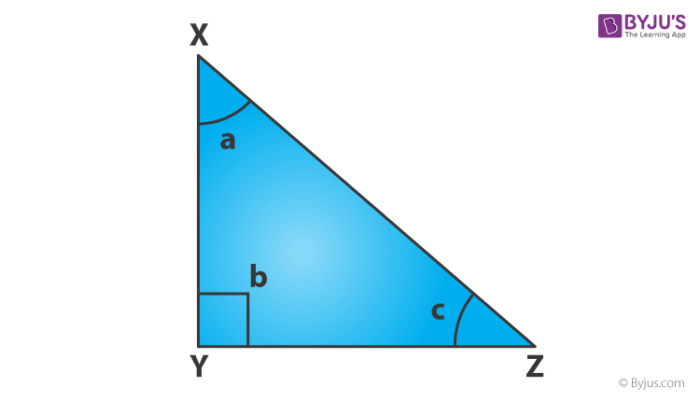What is tan cos and sin?

Tan, cos and sin are three primary trigonometric functions or trigonometric ratios. Tan, cos and sin are abbreviations of tangent, cosine and sine respectively.

Definitions of Sin, Cos and Tan:

In a right-angled triangle,

“Sine function is the ratio of opposite side of angle and hypotenuse”

“Cosine function is the ratio of adjacent of angle and hypotenuse”

“Tangent function is the ratio of opposite side and adjacent side”

Suppose, XYZ is a right angled triangle as per given in the below diagram:Now, we can find the value of sin, cos and tan with respect to angle c, such as:

Sin c = XY/XZ

Cos c = YZ/XZ

Tan c = XY/YZ(0)(1)